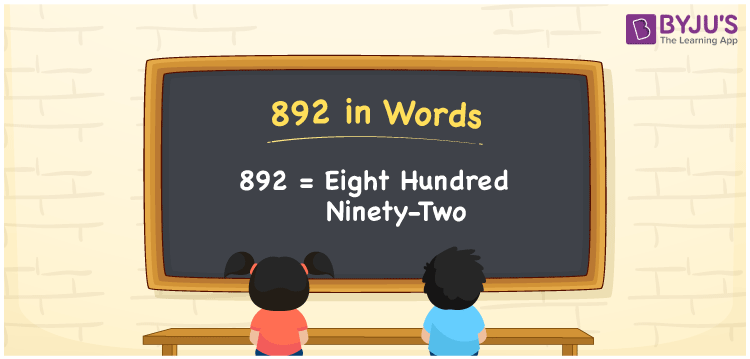# 892 in Words

892 in words is written as Eight hundred ninety-two. In both the International System of Numerals and the Indian System of Numerals, 892 is written as Eight hundred ninety-two. The number 892 is a Cardinal Number as it represents some quantity. For example, the “cost of the dress is rupees 892”.

 892 in Words Eight hundred ninety-two Eight hundred ninety-two in Number 892

## 892 in English Words

We write 892 in English Words using the letters of the English alphabet. Therefore, we read 892 in English as “Eight hundred ninety-two”.## How to Write 892 in Words?

To write 892 in words, we shall use the place value chart. In the place value chart, write 8 in the hundreds, 9 in the tens, and 2 in the ones, respectively. Now let us make a place value chart to write the number 892 in words.

 Hundreds Tens Ones 8 9 2

Thus, we can write the expanded form as

8 × Hundred + 9 × Ten + 2 × One

= 8 × 100 + 9 × 10 + 2 × 1

= 800 + 90 + 2

= 892

= Eight hundred ninety-two.

892 is a natural number, the successor of 891 and the predecessor of 893.

892 in words – Eight hundred ninety-two

• Is 892 an odd number? – No
• Is 892 an even number? – Yes
• Is 892 a perfect square number? – No
• Is 892 a perfect cube number? – No
• Is 892 a prime number? – No
• Is 892 a composite number? – Yes

## Frequently Asked Questions on 892 in Words

Q1

### How to write 892 in words?

892 in words is written as Eight hundred ninety-two.
Q2

### How to write 892 in the International and Indian System of Numerals?

In both, the system of numerals, 892 in words, is written as Eight hundred ninety-two.
Q3

### What is the successor of 892?

The successor of 892 is 893.